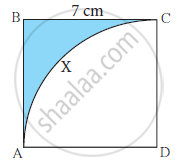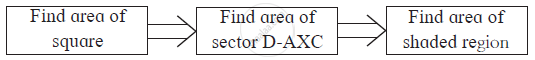Advertisement Remove all ads

# Side of Square Abcd is 7 Cm. with D as the Centre and Da as Radius, Arc Axc is Drawn.Find the Area of the Shaded Region with the Help of the Following Flow Chart . - GeometrySide of square ABCD is 7 cm. With D as the centre and DA as radius, arc AXC is drawn.Find the area of the shaded region with the help of the following flow chart .Advertisement Remove all ads

#### Solution

Area of square ABCD = side2
= 7
= 49 cm2
Sector D-AXC = θ/360 × πr2
= 90/360 × 22/7 × 7^2
= 1/4 × 22/7 × 7 × 7
= 154/4
= 38.5 cm^2
∴ Area of shaded portion = 49 - 38.5
= 10.5  cm^2

Concept: Theorem - Converse of Tangent at Any Point to the Circle is Perpendicular to the Radius
Is there an error in this question or solution?
Advertisement Remove all ads
Advertisement Remove all ads
Share
Notifications

View all notifications

Forgot password?